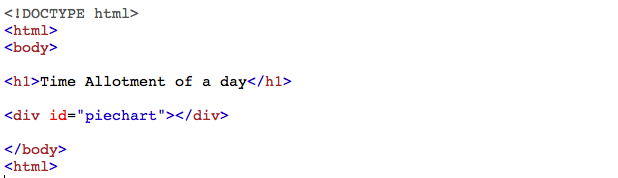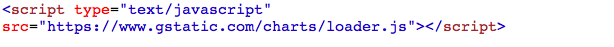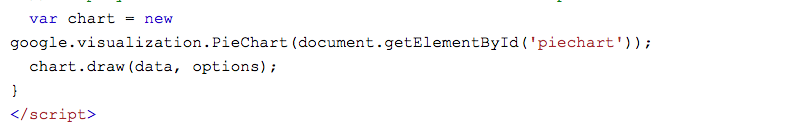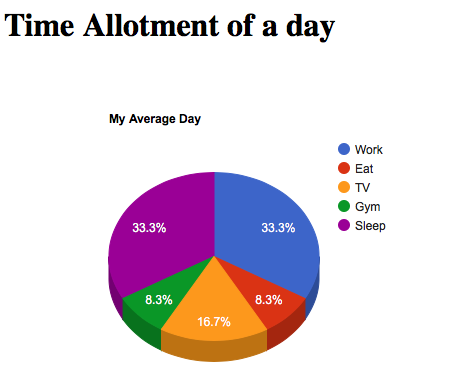Open in App
Not now

• Difficulty Level : Hard
• Last Updated : 21 Oct, 2021

What is a pie chart?
A pie chart (or a circle chart) is a circular statistical graphic which is divided into slices to illustrate numerical proportion. In a pie chart, the arc length of each slice (and consequently its central angle and area), is proportional to the quantity it represents. While it is named for its resemblance to a pie which has been sliced, there are variations on the way it can be presented.

Applications of Pie chart

• Pie charts are generally used to show percentage or proportional data of sales of different items in a business.
• Used in elections to show percentage of votes each party has received.
• Used in surveys to depict no of items in each category.
• Broadly used in database and excel sheets.

Add a “div” element with the id “piechart”:Step 2 : Adding a referenceStep 3 : Add a JavaScript functionExplanation : The below code is used to load Google charts :The below code is used to draw a chart and set the user specific values for each division :The code below is used to display the chart inside the “div” element with id=”piechart” :INPUT :

 ` ` `<``html` `lang``=``"en-US"``> ` `<``body``> ` ` `  `<``h1``>Time Allotment of a day ` ` `  `<``div` `id``=``"piechart"``> ` ` `  `<``script` `type``=``"text/javascript"` ` ``src``=``"https://www.gstatic.com/charts/loader.js"``> ` ` `  `<``script` `type``=``"text/javascript"``> ` `google.charts.load('current', {'packages':['corechart']}); ` `google.charts.setOnLoadCallback(drawChart); ` ` `  `function drawChart() { ` `  ``var data = google.visualization.arrayToDataTable([ ` `  ``['Task', 'Hours per Day'], ` `  ``['Work', 8], ` `  ``['Eat', 2], ` `  ``['TV', 4], ` `  ``['Gym', 2], ` `  ``['Sleep', 8] ` `]); ` `  ``var options = {'title':'My Average Day', 'width':550, 'height':400}; ` `  ``var chart = ` ` ``new google.visualization.PieChart(document.getElementById('piechart')); ` `  ``chart.draw(data, options); ` `} ` ` ` ` `  ` ` ` `

OUTPUT :To get a 3d pie chart, set “is3d” option to true :INPUT :

 ` ` `<``html` `lang``=``"en-US"``> ` `<``body``> ` ` `  `<``h1``>Time Allotment of a day ` ` `  `<``div` `id``=``"piechart"``> ` ` `  `<``script` `type``=``"text/javascript"`  `src``=``"https://www.gstatic.com/charts/loader.js"``> ` ` `  `<``script` `type``=``"text/javascript"``> ` `google.charts.load('current', {'packages':['corechart']}); ` `google.charts.setOnLoadCallback(drawChart); ` ` `  `function drawChart() { ` `  ``var data = google.visualization.arrayToDataTable([ ` `  ``['Task', 'Hours per Day'], ` `  ``['Work', 8], ` `  ``['Eat', 2], ` `  ``['TV', 4], ` `  ``['Gym', 2], ` `  ``['Sleep', 8] ` `]); ` ` `  `  ``var options =  ` `   ``{'title':'My Average Day',  'width':550,'height':400,is3D: true}; ` `   `  `  ``var chart =  ` `  ``new google.visualization.PieChart(document.getElementById('piechart')); ` `  ``chart.draw(data, options); ` `} ` ` ` ` `  ` ` ` `

OUTPUT :My Personal Notes arrow_drop_up
Related Articles